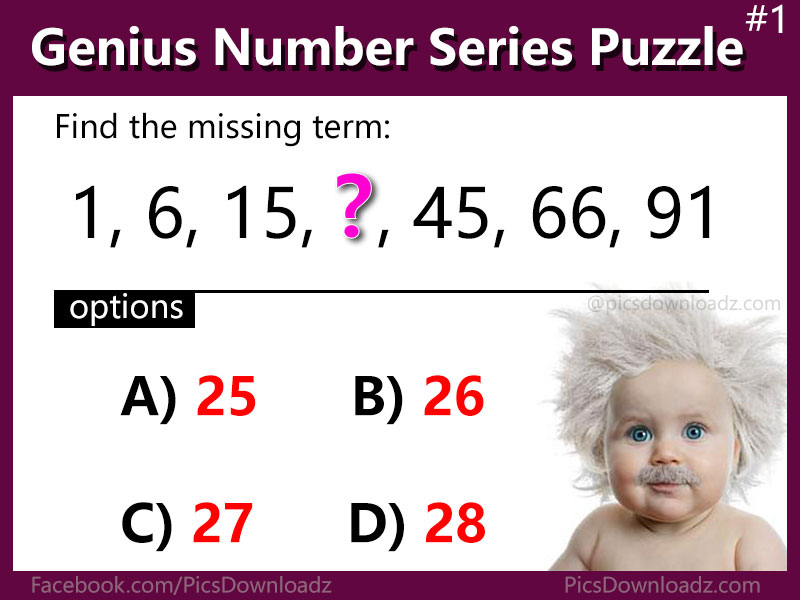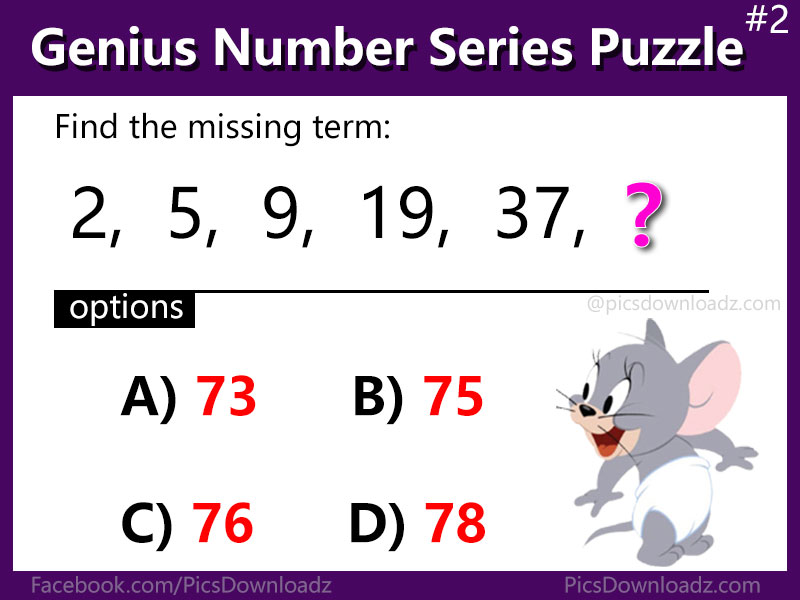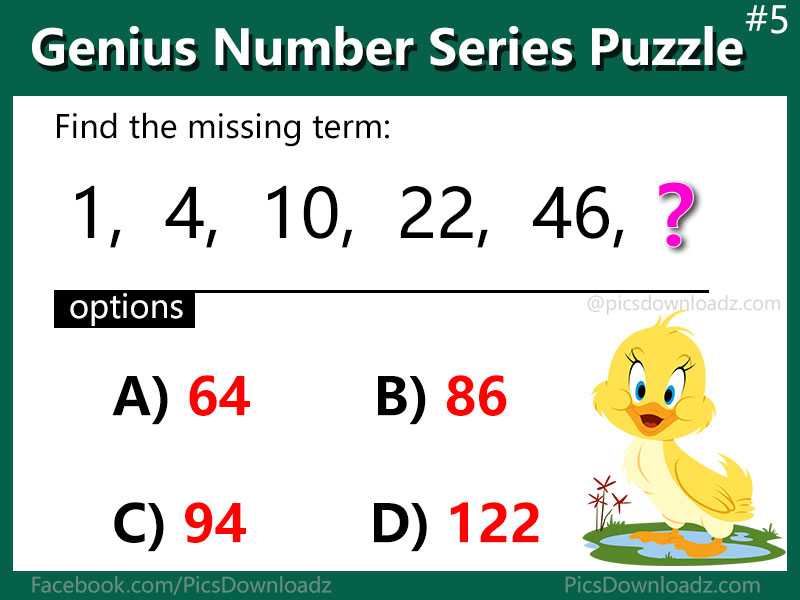This post may contain affiliate links. This means if you click on the link and purchase the item, We will receive an affiliate commission at no extra cost to you. See Our Affiliate Policy for more info.

5 Number Series Puzzles that will blow your mind – Genius Math Puzzles

0
8703

Solve this genius number series math puzzles. 90% will fail to solve these puzzles. Number series puzzle with answer

Hello friends, these question asked in the various competitive exams. These terms follow a certain pattern throughout the series. You have to identify the pattern followed in the series and complete the given series or find the missing term.

Let’s see how’s your reasoning, find which number will replace the question mark and share your answer in the comment section below. Best of luck!

5 Number series Puzzle Question:

#1 Find the missing number

⇒ 1, 6, 15, ?, 45, 66, 91

Options: a) 25,  b) 26,  c) 27 and d) 28#2 Find the last digits

⇒ 2, 5, 9, 19, 37, ?

Options: a) 73, b) 75, c) 76 and d) 78#3 Find the last number

⇒ 6, 13, 25, 51, 101, ?

Options: a) 201, b) 202, c) 203, and d) 205#4 Find next number

⇒ 2, 3, 8, 27, 112, ?

Options: a) 226, b) 339, c) 452, and d) 565#5 Which number replace the question mark

⇒ 1, 4, 10, 22, 46, ?

Options: a) 64, b) 86, c) 94, and d) 122Got it? Let’s see, how many puzzles you have cracked. Comment your answer below or keep scrolling for correct answer and solution for these puzzles.

.

A

N

S

W

E

R

.

#1 – d) 28

#2 – b) 75

#3 – c) 203

#4 – d) 565

#5 – c) 94

Solution:

#1 Question:

1, 6, 15, ?, 45, 66, 91

The given sequence follows the patterns: +5, +9, + 13, +17, +21, +25, and so on…

So, the missing number is = 15 + 13 = 28

Hence, the answer is d) 28.

#2 Question:

2, 5, 9, 19, 37, ?

Clearly, we have the pattern:

2 x 2 + 1 = 5,

5 x 2 – 1 = 9,

9 x 2 + 1 = 19,

19 x 2 – 1 = 37, so on

Therefore; 37 x 2 + 1 = 75

Hence, the answer is b) 75

#3 Question:

6, 13, 25, 51, 101, ?

The pattern is;

x 2 + 1,

x 2 – 1,

x 2 + 1,

x 2 – 1,

x 2 + 1,

x 2 – 1, so on…

So, the missing term is; 101 x 2 + 1 = 203

Hence, the answer is c) 203

#4 Question:

2, 3, 8, 27, 112, ?

The pattern is:

x 1 + 1,

x 2 + 2,

x 3 + 3,

x 4 + 4,  so on…

So, the missing number is; 112 x 5 + 5 = 565

Hence, the answer is d) 565

#5 Question:

1, 4, 10, 22, 46, ?

The pattern is; +3, +6, +12, +24, so on…

So, the missing term is = 46 + 48 = 94

Therefore, the correct answer is c) 94

Don’t forget to share these puzzle with your friends. Like or follow us on Facebook for more fun and interesting puzzle. You can also join our facebook group if you love these finds of puzzles, the links are given below. Take Care, Enjoy!

Like our Page: